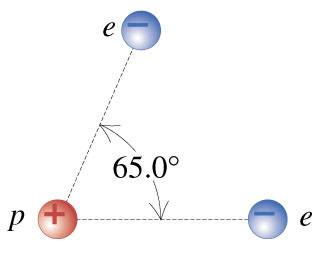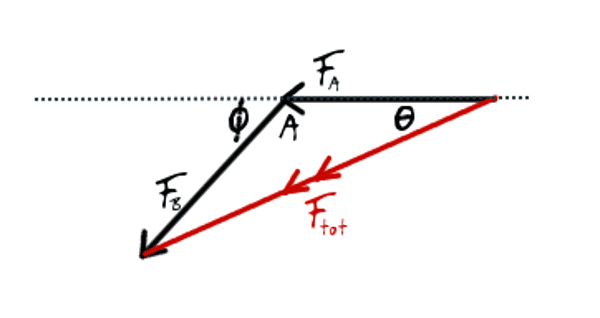# Find the magnitude and of the net electrical force they will exert on the proton.

Mdhiggenz

## Homework Statement

Take a look at this image firstIf two electrons are each 2.50×10−10 from a proton, as shown in the figure, find the magnitude and of the net electrical force they will exert on the proton.

## The Attempt at a Solution

k=1/4πε0

So what I did first was calculate the Electric Force in the x directon

Fx= (k*q^2/r^2)cos(theta)

Fy=( k*q^2/r^2)sin(theta)

Then took the magnitude of the sum √(x)^2+(y)^2

And thought that would give me the answer for part one, where did I go wrong?

Homework Helper
You seem to have left off the cotribution
The force on the proton is the sum of the forces due to the two electrons.
$$F=\frac{ke^2}{r^2}$$ ... for each electron - but the directions are different.
You also need to show the correct direction for the resultant force.

Mdhiggenz
I dont quite understand? Wouldn't me getting the cos / sin be me taking the directions into consideration?

Saitama
I dont quite understand? Wouldn't me getting the cos / sin be me taking the directions into consideration?

I would suggest you not to find the force in a particular direction. Keep everything in variables. Each electron would exert an equal force in magnitude, say F. The resultant force will be $\sqrt{F^2+F^2+2F^2cosθ}$, where θ=65 degrees. Solve the expression, get it into its simplest form i.e factor out F. Plug in the values.

Mdhiggenz
I got the correct answer using your method, but I dont quite understand how you got that.

It would make sense to me if it was √F^2sin∅+F^2cos

But not the √F^2+f^2+2f^2cos∅

can you elaborate on how you achieved that formula?

Thanks

Saitama
I got the correct answer using your method, but I dont quite understand how you got that.

It would make sense to me if it was √F^2sin∅+F^2cos

But not the √F^2+f^2+2f^2cos∅

can you elaborate on how you achieved that formula?

Thanks

That's the formula for finding resultant of two vectors. Don't you know about it?Mdhiggenz
Nope never knew about it but I do now (: is it always cos and never sine?

Saitama
Nope never knew about it but I do now (: is it always cos and never sine?

Yes, it is always cosθ. You should check this out from any physics textbook you have. It should be there in a chapter about "Vectors".Homework Helper
I hate to direct people to memorize equations - the formula is a special case of the cosine rule for a scalene triangle.

To add two vectors, draw them head-to-tail (you should have seen this before) ... the resultant goes from the tail of the first one to the head of the second one. This gives you a triangle. Draw it out and you'll see.

You can also use the sine rule for the same triangle in this case because, since it is an isosceles triangle you know all the interior angles. But this is not generally true.

Homework Helper
I couldn't find a vector-add diagram online that had all the elements I wanted ... so I drew my own:... in the above, we want to do ##\vec{F}_{tot}=\vec{F}_A+\vec{F}_B## - magnitudes and directions are shown in the diagram.

In general ##F_A \neq F_B##:

The cosine rule says that: $$F_{tot}^2 = F_A^2+F_B^2-2F_AF_B\cos(A)$$... notice that ##A=180-\phi## (in degrees) and use the identity: ##\cos(180-\phi)=-\cos(\phi)## to get: $$F_{tot}^2 = F_A^2+F_B^2+2F_AF_B\cos(\phi)$$
Once you've got ##F_{tot}## you can use the sine rule to find ##\theta## - the direction of the resultant. $$\frac{F_{tot}}{\sin(A)} = \frac{F_B}{\sin(\theta)} = \frac{F_A}{\sin(180-A-\theta)}$$

This is not normally any more convenient that working with components ... unless........

In the special case where ##F_A=F_B=F## then ##\theta = \phi/2## and the rule becomes: $$F_{tot}^2 = 2F^2\big ( 1+\cos{\phi} \big )$$ ... which can simplify things a great deal.

In general - learn the sine and cosine rules rather than these vector ones, and get used to drawing the diagrams to guide your thinking.

#### Attachments

Saitama
Nice explanation and nice diagram, Simon Bridge!$$\vec{F}_A = -F_A\hat{\imath}$$ $$\vec{F}_B=-F_B\cos{\phi}\hat{\imath}-F_B\sin(\phi)\hat{\jmath}$$... then I can do: $$\vec{F}_{tot}=\big (-F_A-F_B\cos{\phi}\big ) \hat{\imath}-F_B\sin(\phi)\hat{\jmath}$$